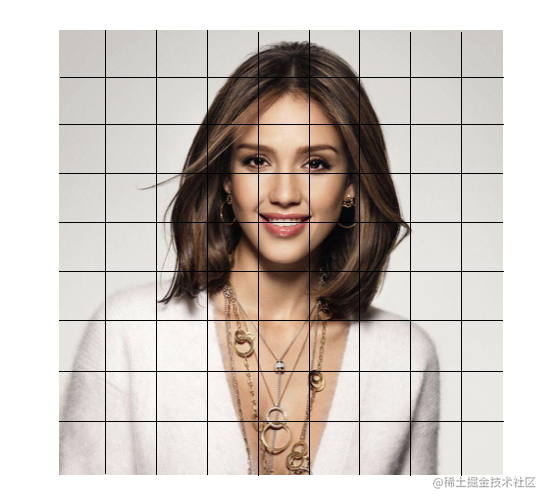OpenGL ES-案例实现灰度滤镜和马赛克滤镜

原理及着色器代码

1、灰度滤镜

• 权值法：处理后的图片比较逼真

• 平均值法：Gray = （R+G+B）/3，处理后的图片比较柔和
• 仅取绿色：Gray = G，这种方式方便简单，且易用

浮点算法代码：

precision highp float;
uniform sampler2D Texture;
varying vec2 TextureCoordsVarying;
//RGB的变换因子，即权重值
const highp vec3 W = vec3(0.2125, 0.7154, 0.0721);

void main(){
//获取对应纹理坐标系下色颜色值
gl_FragColor = vec4(vec3(luminance), 1.0);
}

仅取绿色值：

precision highp float;
uniform sampler2D Texture;
varying vec2 TextureCoordsVarying;

void main(){
//获取对应纹理坐标系下色颜色值

//将RGB全部设置为G，即GRB全部取绿色值
}2、颠倒滤镜

precision highp float;
uniform sampler2D Texture;
varying vec2 TextureCoordsVarying;

void main (void) {
vec4 color = texture2D(Texture, vec2(TextureCoordsVarying.x, 1.0 - TextureCoordsVarying.y));
gl_FragColor = color;
}

3、马赛克滤镜

⻢赛克效果就是把图片的⼀个相当⼤小的区域⽤同一个点的颜色来表示.可以认为是大规模的降低图像的分辨率,而让图像的一些细节隐藏起来。 所以我们只要根据马赛克单元格的宽高计算出图像总的马赛克行数和列数，然后将每个马赛克单元格遍历2次，第一次计算该单元格RGB的平均值，第二次遍历赋颜色值。代码实现:

precision mediump float;
//纹理坐标
varying vec2 TextureCoordsVarying;
//纹理采样器
uniform sampler2D Texture;
//纹理图片size
const vec2 TexSize = vec2(500.0, 500.0);
//马赛克size
const vec2 mosaicSize = vec2(16.0, 16.0);

void main()
{
//计算图像的实际位置
vec2 intXY = vec2(TextureCoordsVarying.x*TexSize.x, TextureCoordsVarying.y*TexSize.y);
// floor (x) 内建函数,返回小于/等于X的最大整数值.
// floor (intXY.x / mosaicSize.x) * mosaicSize.x 计算出一个⼩小⻢赛克的坐标.
vec2 XYMosaic = vec2(floor(intXY.x/mosaicSize.x)*mosaicSize.x, floor(intXY.y/mosaicSize.y)*mosaicSize.y);
//换算回纹理坐标
vec2 UVMosaic = vec2(XYMosaic.x/TexSize.x, XYMosaic.y/TexSize.y);
//获取到马赛克后的纹理坐标的颜色值
vec4 color = texture2D(Texture, UVMosaic);
//将⻢赛克颜色值赋值给gl_FragColor.
gl_FragColor = color;
}

const vec2 mosaicSize = vec2(8.0, 8.0);

4、六边形马赛克

• 偶数行偶数列计算左上中心点和右下中心点即包含六边形的边为/
• 偶数行奇数列计算左下中心点和右上中心点即包含六边形的边为\
• 奇数行偶数列计算左下中心点和右上中心点即包含六边形的边为\
• 奇数行奇数列计算左上中心点和右下中心点即包含六边形的边为/

precision highp float;
uniform sampler2D Texture;
varying vec2 TextureCoordsVarying;
//六边形的边长
const float mosaicSize = 0.03;

void main(){

float length = mosaicSize;
//矩形的高的比例为√3，取值 √3/2 ，也可以直接取√3
float TR = 0.866025;
//矩形的长的比例为3，取值 3/2 = 1.5，也可以直接取3
float TB = 1.5;

//取出纹理坐标
float x = TextureCoordsVarying.x;
float y = TextureCoordsVarying.y;

//根据纹理坐标计算出对应的矩阵坐标
//即 矩阵坐标wx = int（纹理坐标x/ 矩阵长），矩阵长 = TB*len
//即 矩阵坐标wy = int（纹理坐标y/ 矩阵宽），矩阵宽 = TR*len
int wx = int(x / TB / length);
int wy = int(y / TR / length);
vec2 v1, v2, vn;

//判断wx是否为偶数，等价于 wx % 2 == 0
if (wx/2 * 2 == wx) {
if (wy/2 * 2 == wy) {//偶行偶列
//(0,0),(1,1)
v1 = vec2(length * TB * float(wx), length * TR * float(wy));
v2 = vec2(length * TB * float(wx+1), length * TR * float(wy+1));
}else{//偶行奇列
//(0,1),(1,0)
v1 = vec2(length * TB * float(wx), length * TR * float(wy+1));
v2 = vec2(length * TB * float(wx+1), length * TR * float(wy));
}
}else{
if (wy/2 * 2 == wy) {//奇行偶列
//(0,1),(1,0)
v1 = vec2(length * TB * float(wx), length * TR * float(wy+1));
v2 = vec2(length * TB * float(wx+1), length * TR * float(wy));
}else{//奇行奇列
//(0,0),(1,1)
v1 = vec2(length * TB * float(wx), length * TR * float(wy));
v2 = vec2(length * TB * float(wx+1), length * TR * float(wy+1));
}
}
//利用距离公式，计算中心点与当前像素点的距离
float s1 = sqrt(pow(v1.x-x, 2.0) + pow(v1.y-y, 2.0));
float s2 = sqrt(pow(v2.x-x, 2.0) + pow(v2.y-y, 2.0));

//选择距离小的则为六边形的中心点，且获取它的颜色
vn = (s1 < s2) ? v1 : v2;
//获取六边形中心点的颜色值
vec4 color = texture2D(Texture, vn);
gl_FragColor = color;
}

const float mosaicSize = 0.01;

5、三角形马赛克

precision highp float;
uniform sampler2D Texture;
varying vec2 TextureCoordsVarying;

float mosaicSize = 0.03;

void main (void){

float length = mosaicSize;
//矩形的高的比例为√3，取值 √3/2 ，也可以直接取√3
float TR = 0.866025;
//矩形的长的比例为3，取值 3/2 = 1.5，也可以直接取3
float TB = 1.5;

//取出纹理坐标
float x = TextureCoordsVarying.x;
float y = TextureCoordsVarying.y;

//根据纹理坐标计算出对应的矩阵坐标
//即 矩阵坐标wx = int（纹理坐标x/ 矩阵长），矩阵长 = TB*len
//即 矩阵坐标wy = int（纹理坐标y/ 矩阵宽），矩阵宽 = TR*len
int wx = int(x / TB / length);
int wy = int(y / TR / length);
vec2 v1, v2, vn;

//判断wx是否为偶数，等价于 wx % 2 == 0
if (wx/2 * 2 == wx) {
if (wy/2 * 2 == wy) {//偶行偶列
//(0,0),(1,1)
v1 = vec2(length * TB * float(wx), length * TR * float(wy));
v2 = vec2(length * TB * float(wx+1), length * TR * float(wy+1));
}else{//偶行奇列
//(0,1),(1,0)
v1 = vec2(length * TB * float(wx), length * TR * float(wy+1));
v2 = vec2(length * TB * float(wx+1), length * TR * float(wy));
}
}else{
if (wy/2 * 2 == wy) {//奇行偶列
//(0,1),(1,0)
v1 = vec2(length * TB * float(wx), length * TR * float(wy+1));
v2 = vec2(length * TB * float(wx+1), length * TR * float(wy));
}else{//奇行奇列
//(0,0),(1,1)
v1 = vec2(length * TB * float(wx), length * TR * float(wy));
v2 = vec2(length * TB * float(wx+1), length * TR * float(wy+1));
}
}
//利用距离公式，计算中心点与当前像素点的距离
float s1 = sqrt(pow(v1.x-x, 2.0) + pow(v1.y-y, 2.0));
float s2 = sqrt(pow(v2.x-x, 2.0) + pow(v2.y-y, 2.0));

//选择距离小的则为六边形的中心点，且获取它的颜色
vn = (s1 < s2) ? v1 : v2;
//获取六边形中心点的颜色值
vec4 mid = texture2D(Texture, vn);

//获取像素点与中心点的角度
float a = atan((x-vn.x)/(y-vn.y));

//判断夹角，属于哪个三角形，则获取哪个三角形的中心点坐标
vec2 area1 = vec2(vn.x, vn.y - mosaicSize * TR / 2.0);
vec2 area2 = vec2(vn.x + mosaicSize / 2.0, vn.y - mosaicSize * TR / 2.0);
vec2 area3 = vec2(vn.x + mosaicSize / 2.0, vn.y + mosaicSize * TR / 2.0);
vec2 area4 = vec2(vn.x, vn.y + mosaicSize * TR / 2.0);
vec2 area5 = vec2(vn.x - mosaicSize / 2.0, vn.y + mosaicSize * TR / 2.0);
vec2 area6 = vec2(vn.x - mosaicSize / 2.0, vn.y - mosaicSize * TR / 2.0);

if (a >= PI6 && a < PI6 * 3.0) {
vn = area1;
}else if (a >= PI6 * 3.0 && a < PI6 * 5.0){
vn = area2;
}else if ((a >= PI6 * 5.0 && a <= PI6 * 6.0) || (a < -PI6 * 5.0 && a > -PI6 * 6.0)){
vn = area3;
}else if (a < -PI6 * 3.0 && a >= -PI6 * 5.0){
vn = area4;
}else if (a <= -PI6 && a > -PI6 * 3.0){
vn = area5;
}else if (a > -PI6 && a < PI6){
vn = area6;
}
//获取对应三角形重心的颜色值
vec4 color = texture2D(Texture, vn);
// 将颜色值填充到片元着色器内置变量gl_FragColor
gl_FragColor = color;

}

const float mosaicSize = 0.01;

iOS

iOS